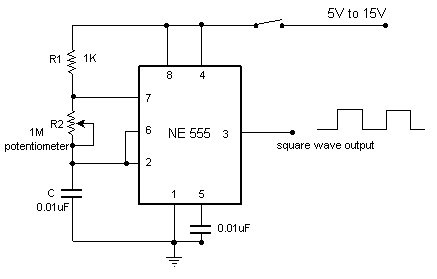# Simple variable frequency oscillator Electronic Schematics > Oscillators and timers > Simple variable frequency oscillatorThis is a very simple circuit utilising a 555 timer IC to generate square wave of frequency that can be adjusted by a potentiometer.

With values given the frequency can be adjusted from a few Hz to several Khz.
To get very low frequencies replace the 0.01uF capacitor with a higher value.

The formula to calculate the frequency is given by:

1/f = 0.69 * C * ( R1 + 2*R2)

The duty cycle is given by:

% duty cycle = 100*(R1+R2)/(R1+ 2*R2)

In order to ensure a 50% (approx.) duty ratio, R1 should be very small when compared to R2. But R1 should be no smaller than 1K.
A good choice would be, R1 in kilohms and R2 in megaohms. You can then select C to fix the range of frequencies.

Title: Simple variable frequency oscillator
electronic circuit
Source: www.electronic-circuits-diagrams.com
Published on: 2005-02-01
Print version: